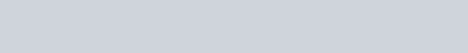As an electron makes a transition from an excited state
Question:

As an electron makes a transition from an excited state to the ground state of a hydrogen – like atom/ion :

1. kinetic energy decreases, potential energy increases but total energy remains same

2. kinetic energy and total energy decrease but potential energy increases

3. its kinetic energy increases but potential energy and total energy decreases

4. kinetic energy, potential energy and total energy decrease.

Correct Option: , 3

Solution: